## Three-dimensional model of three-point bending

As the last and computationally most demanding example, the three-point bending test is simulated using a three-dimensional model. The geometry and boundary conditions are shown in Fig. 6a. The finite element mesh is generated using T3D . It contains 6461 nodes and 33158 constant-strain tetrahedral elements; see Fig. 6b. The constitutive properties are set to: Young's modulus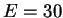GPa, Poisson's ratio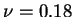, tensile strength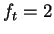MPa, strain controling the softening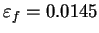, and nonlocal interaction radius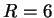mm.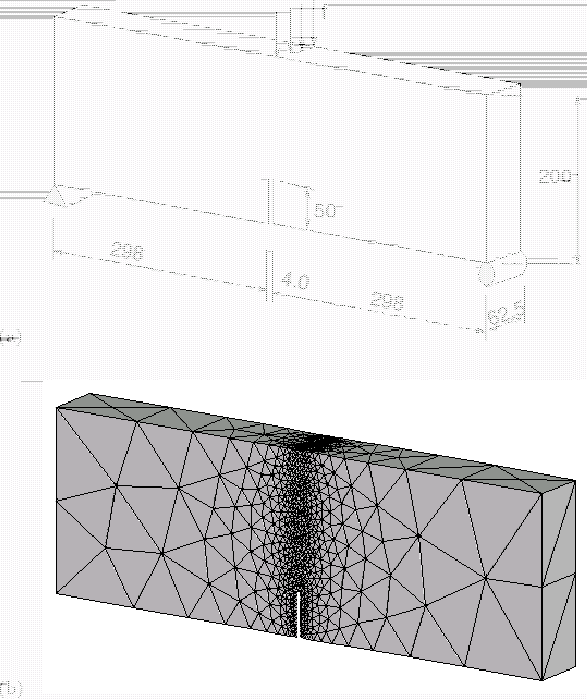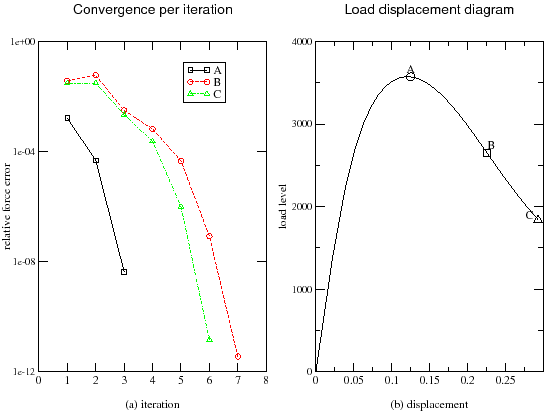Table 7: Three-point bending test in 3D: total execution times
 SSM-10 TSM TSM, GMRES(ILUT) Total time 27h:37m:21s 136h:40m:56s 15h:23m:30s

Solution strategies based on the SSM and TSM with either direct or iterative solvers are exploited. The ESM would again lead to an excessive number of iterations. The TSM converges within 3 to 7 iterations; see Fig. 7a. The basic information about the solution times in a high-accuracy analysis is provided in Table 7. The assembly time for SSM-10 is 1.3 seconds, for TSM with the direct solver using skyline storage between 6.1 and 78 seconds, and for TSM with the iterative solver using compressed row storage between 8.1 and 84.3 seconds. The factorization time for SSM-10 is 201.1 seconds, and for TSM with direct solver between 620 and 2260 seconds. The solution time for the iterative GMRES solver is between 16.8 and 37.3 seconds plus the time needed for preconditioning, which is between 7.4 and 119.4 seconds. Due to the high bandwidth of the tangent stiffness matrix, emphasized by the three-dimensional character of the mesh, the direct solver becomes quite expensive. With a direct solver, the TSM strategy is about 5 times slower than the SSM strategy. However, the GMRES iterative solver with preconditioning by incompletedecomposition leads to a dramatic acceleration and reduces the total execution time by one order of magnitude. This is due to the fact that the solver can profit from the high sparsity of the stiffness matrix. The iterative solver is not suitable for the secant stiffness matrix, because the number of iterations is relatively high and the iterative solver cannot take advantage of the fact that the stiffness matrix remains the same during a certain number of iterations. Thus, the best results are obtained with the tangent stiffness and iterative solver, which illustrates the potential of the proposed method in large three-dimensional problems.